Next: Non-Cartesian Coordinates Up: Cartesian Tensors Previous: Isotropic Tensors

# Exercises

1. Show that a general second-order tensor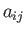can be decomposed into three tensors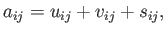where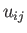is symmetric (i.e.,) and traceless (i.e.,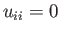),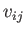is isotropic, and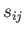only has three independent components.

2. Use tensor methods to establish the following vector identities:
1.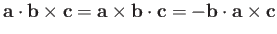.
2..
3.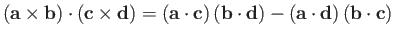.
4.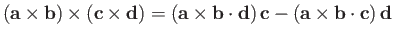.
5.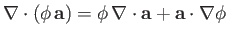.
6.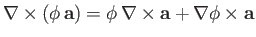.
7.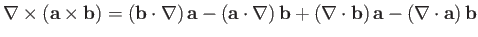.
8..
9.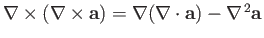.
Here,, and.

3. A quadric surface has an equation of the form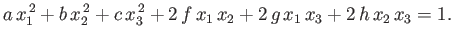Show that the coefficients in the previous expression transform under rotation of the coordinate axes like the components of a symmetric second-order tensor. Hence, demonstrate that the equation for the surface can be written in the form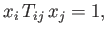where theare the components of the aforementioned tensor.

4. The determinant of a second-order tensoris defined1. Show that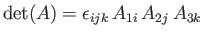is an alternative, and entirely equivalent, definition.
2. Demonstrate that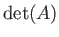is invariant under rotation of the coordinate axes.
3. Suppose that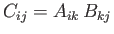. Show that5. Ifthen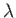and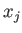are said to be eigenvalues and eigenvectors of the second-order tensor, respectively. The eigenvalues ofare calculated by solving the related homogeneous matrix equationNow, it is a standard result in linear algebra that an equation of the previous form only has a non-trivial solution when (Riley 1974)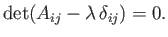Demonstrate that the eigenvalues ofsatisfy the cubic polynomial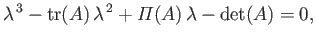whereand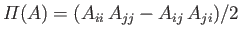. Hence, deduce thatpossesses three eigenvalues--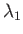,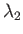, and(say). Moreover, show that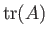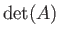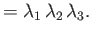6. Suppose thatis a (real) symmetric second-order tensor: that is,.
1. Demonstrate that the eigenvalues ofare all real, and that the eigenvectors can be chosen to be real.
2. Show that eigenvectors ofcorresponding to different eigenvalues are orthogonal to one another. Hence, deduce that the three eigenvectors ofare, or can be chosen to be, mutually orthogonal.
3. Demonstrate thattakes the diagonal form(no sum) in a Cartesian coordinate system in which the coordinate axes are each parallel to one of the eigenvectors.

7. In an isotropic elastic medium under stress, the displacement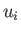satisfies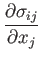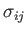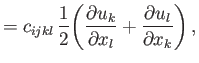where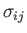is the stress tensor (note that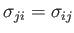),the mass density (which is a uniform constant), and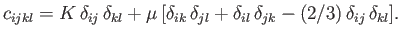the isotropic stiffness tensor. Here,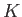and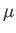are the bulk modulus and shear modulus of the medium, respectively. Show that the divergence and the curl of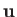both satisfy wave equations. Furthermore, demonstrate that the characteristic wave velocities of the divergence and curl waves are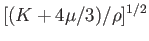and, respectively.Next: Non-Cartesian Coordinates Up: Cartesian Tensors Previous: Isotropic Tensors
Richard Fitzpatrick 2016-03-31